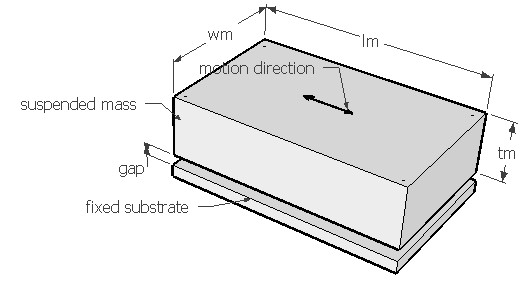SLDSUS,lm,wm,tm,fre,gap,den,sel
Slide film damping of a suspended mass

lm          length of the mass in µm
wm        width of the mass in µm
tm          thickness of mass in µm
fre         natural frequency of vibration of the microsuspension in Hz
gap        air gap distance in µm
den        density of the material of the beam in kg/m3
sel          number denoting the selected result.
Use 1 for quality factor, 2 for coefficient of damping force and 3 for damping ratioNotes

Air film damping plays a significant part in the design of micro electro mechanical devices. If a stationary surface is placed in close proximity to a mass or another surface which is allowed to move parallel to its plane, a velocity gradient will develop in the air film in the gap. The work done in this process would reduce the energy of the suspension and thereby the air acts as a damper and the process is called slide film damping. When the frequency of oscillation of the mass is small and the gap is small, the velocity gradient can be assumed to be constant and such flow is called Couette flow. This is the type of slide film damping that predominates in most MEMS devices where small gaps and low frequency are involved.

Resistive shearing force on the moving surface from gas film can be obtained from Navier-Stokes equation. The coefficient of damping force can be calculated from this provided the air gap is known. If free vibration frequency of the microsuspension is known, the damping ratio can be calculated from it for that particular mode of vibration. The natural vibration frequency can be estimated under Vibration > Free vibration. The vibration mode should be such that the movement of the suspended mass is parallel to the fixed substrate as shown in the figure.

This design form can be used to estimate the coefficient of damping force for the given air gap and plate design. Damping ratio is calculated for the given vibration frequency. It is assumed that the gap is small and the vibration frequency is small such that Couette flow model is valid. There is slide film damping on top of the suspended mass where it is far away from any other surface. This damping though is assumed to be much smaller than the Couette flow damping and is considered negligible.  The fixed substrate is considered only on bottom side of the mass. If there is another fixed substrate near the top surface of the mass, then damping due to that can be estimated separately and the effective damping coefficient will be a sum of the two. The quality factor is calculated from the damping ratio which is quite high due to low damping.

The plot shows the variation of damping ratio with the air gap thickness. Using the crosshair tool, the damping ratio for any air gap thickness upto 10µm can be estimated.

Assumptions

-The default material is Silicon and ambient is air with viscosity of 1.8e-5 Pa.sec at 20°C.
-The free vibration mode leads to slide film damping.
-The frequency of vibration is low and air gap is small.
-Slide film damping for large gaps due to Stokes flow is assumed to be negligible.
-The mass of the beam is negligible.
-The damping caused by the beam area is negligible.